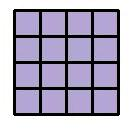### Squares

When a number is multiplied by itself, it is called a square:

 12 = 1 × 1 = 1 32 = 3 × 3 = 922 = 2 × 2 = 4 42 = 4 × 4 = 16to: